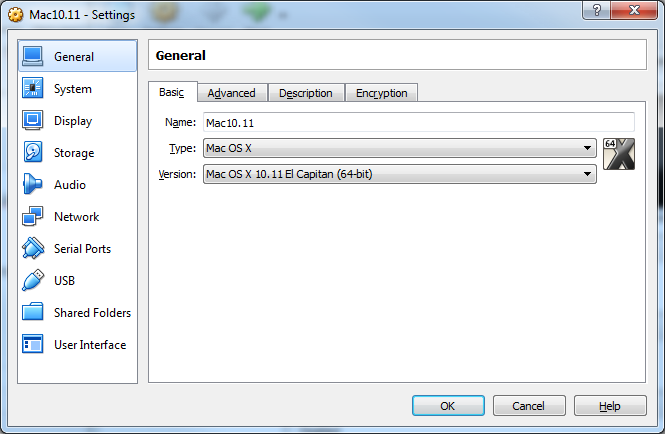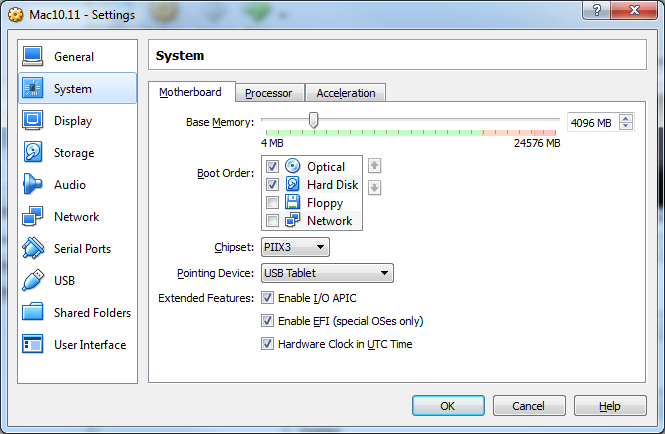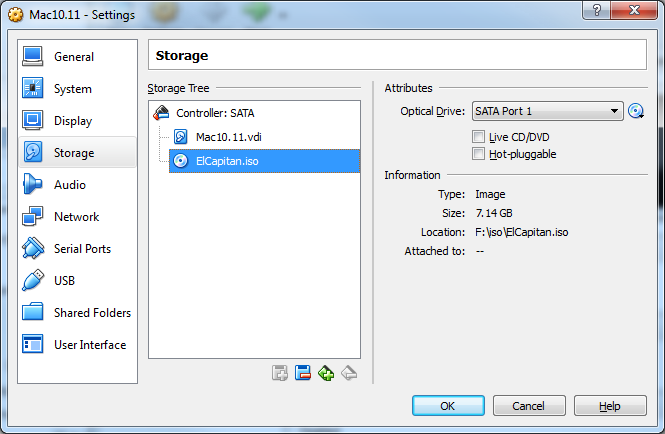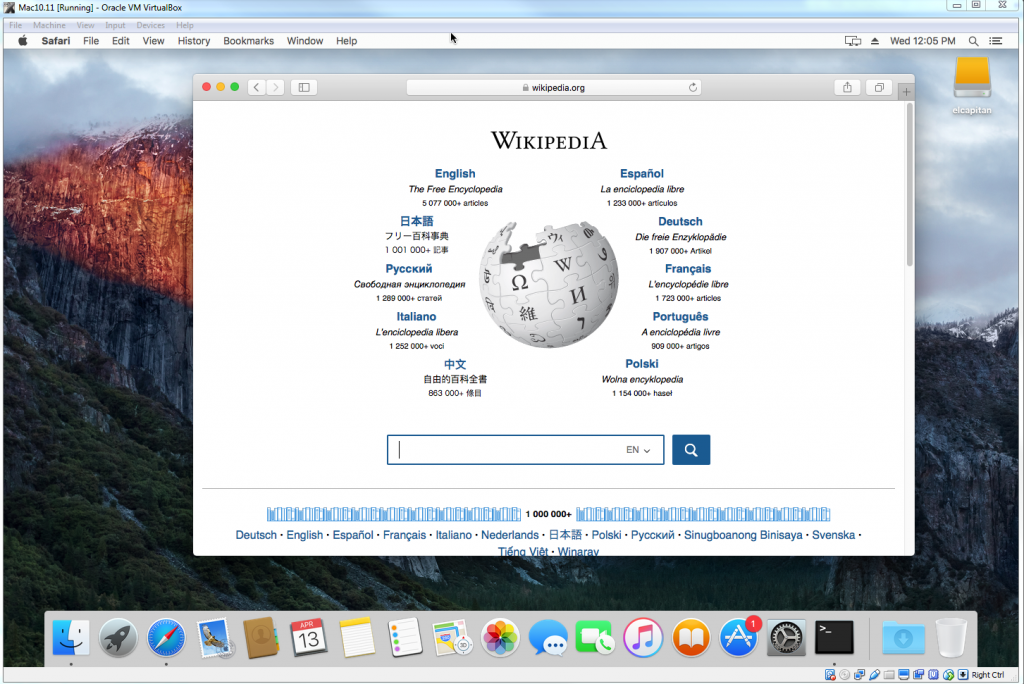# lapack sgetrs tutorial

I have a problem to solving system linear equation Ax=b using lapack. I am planned to use sgetrs subroutine in lapack to solving system linear equation Ax=b.

sgetrs descriptions is a subroutine to Solves a system of linear equations with an LU-factored square coefficient matrix, with multiple right-hand sides. So, we need to process our data with LU-factored before use sgetrs lapack.

I have create a C/C++ code to give tutorial how to call sgetrs lapack from C/C++ code. I have compared the result from this sgetrs lapack with Matlab and give the same result. Please check this code below (call sgetrs lapack from C/C++) :

We can compile this code (call sgetrs lapack from C/C++) using command :

Sample output after running this code (call sgetrs lapack from C/C++) :

# gausswin source code

I want to create gaussian window program in C/C++. I have checked at Matlab and they give gausswin function to create gaussian window. I have checked gausswin Matlab source code and convert that code to C/C++.

The coefficients of a Gaussian window are computed from the following equation:where –(N – 1)/2 ≤ n ≤ (N – 1)/2 and α is inversely proportional to the standard deviation, σ, of a Gaussian random variable. The exact correspondence with the standard deviation of a Gaussian probability density function is σ = (N – 1)/(2α).

Compile this gausswin.c (gaussian window source code) using command :

Show gaussian window program manual using command :

Sample command how to run gaussian window program

I have compare the result from my gaussian window program and show same result compared with gausswin Matlab.

# VirtualBox Install El Capitan

I am very interesting to install Mac OS in my desktop computer using VirtualBox. I have created steps how to install Mac OS in VirtualBox. But, you need existing Mac OS to create bootable Mac OS.

I want to install Mac El Capitan (10.11) in my VirtualBox. I dont have fast internet connection. So, I am search an iso Mac El Capitan over internet.

2.  The Mac iso image from link above not bootable (El Capitan.dmg). So, we need to convert Mac not bootable to iso bootable. I have convert *.dmg file to iso bootable iso using this script (you need to run this script from your existing Mac).

3.  Using above script, we get ElCaption.iso. This file is bootable iso image needed to install El Capiton (Mac 10.11) in VirtualBox.

4. Open VirtualBox, create a new Mac project. I have created project using this setting. If you want change project Name, dont forget to edit text command in next steps too.5.  Close VirtualBox. Choose a Mac Model similar to your host system. Open your console and type this text. I have choose “MacBookPro11,3” in my desktop computer.

6.  Optional- For some host systems eg those with Haswell CPUs, you might have to spoof an older CPU to avoid VirtualBox errors.  You can try from one of the following if this happens:

7. Open VirtualBox and run our Mac10.11 Project. I think you already know how to install Mac in your computer. If not, you can search over internet.

8. When you success install Mac In your VirtualBox, you can change default screen resolution using this command :

9. Adding serials and other SMBIOS details for the System Information Screen (optional)

I have success using this steps and this is how look Mac 10.11 in VirtualBoxSource :
http://www.insanelymac.com/forum/topic/309654-run-vanilla-os-x-el-capitan-yosemite-or-mavericks-in-virtualbox-5010-on-a-windows-host/

# Matlab Data Precision Problem

I have created a simple computation using Matlab using command :

Nothing wrong with the result. But, try to change data precision format and try to retype command above. we will get Matlab data precision problem from this simple computation.

We get the wrong result. I hope can get 71.5000 from above command. This precision can be bigger problem if we use the result to create a variable for next computation like below :

Manual computation will get result 72.

I dont know why Matlab can not solve this simple computation with the right precision (Matlab Data Precision Problem).  Sometime, we need to add a variable like 0.5 to get the right result.

I am testing this computation using Matlab 2013a and Matlab 2015a ad still get the same result (Matlab data precision problem). I hope Matlab can solve this problem in the next release.